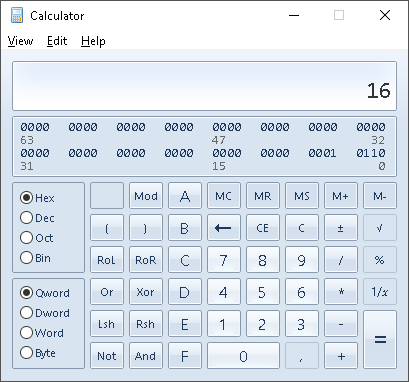# Binary, decimal and hex

If you are not familiar with binary numbers and bits please remember, that they always start with the most significant bit first. This is not special to the RFzero or Arduino but a general principle. This is in fact similar to the decimal system, where we also start with the most significant digit first, e.g. 7042 is seven thousands, zero hundreds, four tens and two ones which is equal to 7 • 1000 + 0 • 100 + 4 • 10 + 2 • 1 = 7 • 103 + 0 • 102 + 4 • 101 + 2 • 100.

Please remember that xy is the same as x-value multiplied by itself y-number of times, e.g. 103 = 10 • 10 • 10 = 1000.

If we have the binary number 01000101 we can do the same principle break down as we did with the 7042 decimal number above. But instead of the base being ten (10) in the decimal world, it is two (2) in the binary world, i.e. 01000101 can be expressed as 0 • 27 + 1 • 26 + 0 • 25 + 0 • 24 + 0 • 23 + 1 • 22 + 0 • 21 + 1 • 10 = zero one-hundred-and-twenty-eights, one sixty-fours, zero thirty-twos, zero sixteens, zero eights, one fourths, zero twos and one ones.

Oh, please remember always to multiply/divide before adding/subtracting! Otherwise your old math teacher will be after you.

Binary, decimal and hexadecimal conversion table.

BinDecHexBinDecHexBinDecHexBinDecHex
000000010044100088110012C
000111010155100199110113D
001022011066101010A111014E
001133011177101111B111115F

Converting binary bits to hex is easier than you may think. Simply take four bits starting from the right, and convert them to hex, then take the next group of four bits moving left and so on, until there are no more bits left, see the table below.

Examples of converting binary numbers to hexadecimal.

DecimalBinaryGroups of fourHexHex notation
0000000000000 00000 00x00
22000101100001 01101 60x16
69010001010100 01014 50x45
255111111111111 1111F F0xFF

If there is less than four bits just imagine that the missing bits to the left are zeros, e.g. 110100 => 00110100.

You may also use a program to do the conversion for you. In Windows and Mac OS there are programs called Calculator, that can be changed to Programmer mode. In Linux there is to many to mention.

Example of the Windows Calculator in Programmer mode (Menu | View | Programmer).Generally when programming in C and variants

• Binary numbers must be prefixed 0b, e.g. 0b10101111
• Decimal numbers are often not prefixed but can be 0d, e.g. 0d7042
• Hexadecimal numbers must be prefixed 0x, e.g. 0x1F
• If a number begins with zero (0) it is an octal number, which is not used very often. But take care not to write e.g. 01011111, and think it is a binary number because it isn’t